# A simple model of nonlinear growth

## 2003-10-01

Part of the challenge in nonlinear regression is to choose the correct form of the nonlinear relationship. Part of this is intuition, part of this is understanding some basic mathematics, and part of it is just trial and error.

A very simple growth model

The simplest model of growth uses the exponential function. If you are trying to describe growth that eventually levels off, then the formula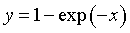{width=“130” height=“33”}

is a good place to start. You can generate this formula using differential equations that assume that the rate of growth is proportional to the amount of growth left until full maturity.

Here’s a graph of this formula.{width=“298” height=“230”}

Notice that when x equals 1, about two-thirds of the growth has already occurred and that when x equals 3, about 95% of the growth has occurred.

Allowing for changes in the growth rate

We can change the rate of growth by modifying the equation to{width=“138” height=“33”}

Here’s what the graph looks like for three values of b.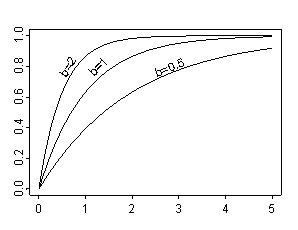{width=“298” height=“230”}

Notice that a larger value of b implies faster growth. As a rough rule of thumb, about 2/3 of the growth will occur at x=1/b and about 95% of the growth will occur by x=3/b. For example, when b=0.5, about two-thirds of the growth has occurred by x=2 and 95% has occurred by x=6 (which is off the scale of this graph).

Allowing for different starting and ending values

We need to make two more modifications to the formula, though. First, we need to account for a full grown size that is usually a value different from 1. Second, we need to account for a starting size that is usually larger than 0. These requirements modify the equation to{width=“155” height=“33”}

With this equation, the full-grown size is represented by c, and the initial size at x=0 is represented by c-a. Here’s an example of this graph with c=0.9, a=0.7, and b=2.{width=“298” height=“230”}

You can change the values of c, a, and b to illustrate a variety of different growth patterns. For example, here are three growth curves that have different initial sizes, but which all reach the same full grown size.{width=“298” height=“230”}

Here are three growth curves that start at the same initial size but which reach different full grown sizes.{width=“298” height=“230”}

An example using real data

Here’s some data on the growth of a child who was born prematurely.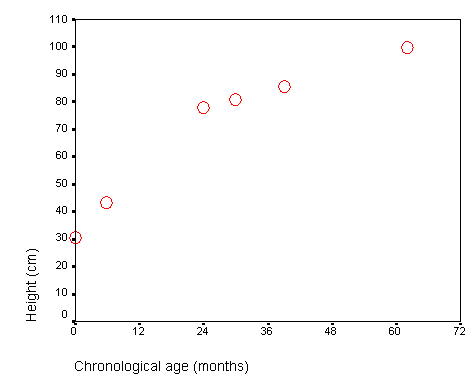{width=“476” height=“389”}

When you ask SPSS to fit a nonlinear regression model (select ANALYZE | REGRESSION | NONLINEAR), your dialog box will look like this: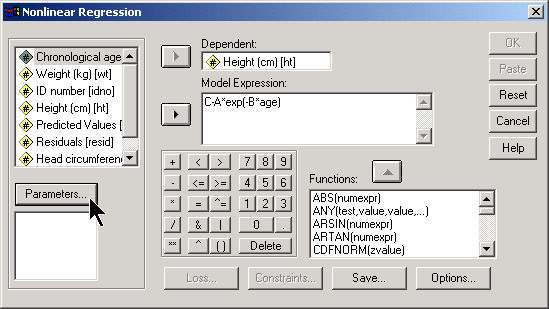{width=“549” height=“309”}

Notice that I specified the nonlinear model as “C-Aexp(-Bage)” and used upper case for the values C, A, and B. This makes it easier to distinguish between these parameters and the variables.

Now you have to provide SPSS with some starting values. They don’t have to be too accurate, but they need to be in the right ball park. Click on the PARAMETERS button to provide starting values.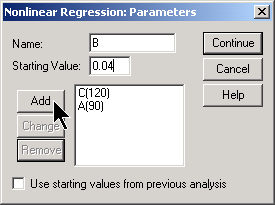{width=“275” height=“205”}

If you look at the graph the we drew for the data, it looks like it might level off around 120, so that’s a good starting value for C. The initial size is around 30. Since C-A represents the initial size, a value of 90 would be a good starting value for A. Finally, it looks like more than half of the growth occurs in the first 24 months. So a good starting value for B would be 1/24, which I rounded to 0.04.

After you have added all your starting values, click on the continue button. Here is what the dialog box looks like now.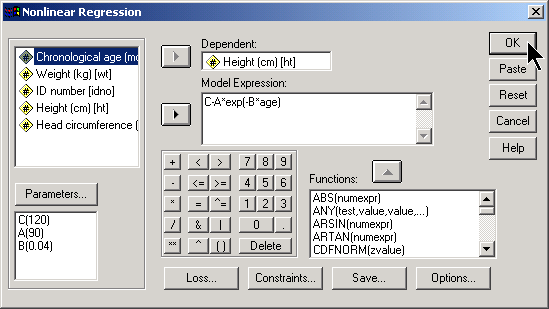{width=“549” height=“309”}

If you want to compute predicted values and residuals, click on the SAVE button. Otherwise, click on OK to run your nonlinear regression model. Here is a portion of the output that SPSS produced.{width=“533” height=“309”}

Our model shows an initial size of about 30 cm, a full-grown size of about 107 cm and a growth rate value of 0.037. Also notice how wide the confidence intervals are for each of the parameters. This is not too surprising, since our sample size is so small.

Be careful with this data. The full grown size represents an extrapolation beyond the range of the data (most children continue to grow quite a bit beyond 72 months). In fact, it looks like the estimate of full grown size is a serious underestimate (107 cm is about 42 inches). Perhaps this underestimate is also telling me that my choice for a nonlinear model is not a good one.

Here is the original data (red circles) and the values predicted by the nonlinear regression model (blue line).{width=“476” height=“389”}

There is a good level of agreement between the growth model and the data.

You can find an earlier version of this page on my original website.